# 6.4: Kinetic Molecular Theory (Overview)

•• Contributed by Stephen Lower
• Professor Emeritus (Chemistry) at Simon Fraser University

Skills to Develop

Make sure you thoroughly understand the following essential ideas which are presented below. It is especially important that you know the principal assumptions of the kinetic-molecular theory. These can be divided into those that refer to the nature of the molecules themselves, and those that describe the nature of their motions:

• The molecules - Negligible volume, absence of inermolecular attractions (think of them as very hard, "non-sticky" objects.)
• Their motions - Completely random in direction, in straight lines only (this is a consequence of their lack of attractions), average velocities proportional to the absolute temperature..
• The idea that random motions of individual molecules can result in non-random (directed) movement of the gas as a whole is one of the most important concepts of chemistry, exemplified here as the principle of diffusion.
• In most courses you will be expected to know and be able to use (or misuse!) Graham's law.

The properties such as temperature, pressure, and volume, together with others dependent on them (density, thermal conductivity, etc.) are known as macroscopic properties of matter; these are properties that can be observed in bulk matter, without reference to its underlying structure or molecular nature. By the late 19th century the atomic theory of matter was sufficiently well accepted that scientists began to relate these macroscopic properties to the behavior of the individual molecules, which are described by the microscopic properties of matter. The outcome of this effort was the kinetic molecular theory of gases. This theory applies strictly only to a hypothetical substance known as an ideal gas; we will see, however, that under many conditions it describes the behavior of real gases at ordinary temperatures and pressures quite accurately, and serves as the starting point for dealing with more complicated states of matter.

## The basic ideas of kinetic-molecular theory

The "kinetic-molecular theory of gases" may sound rather imposing, but it is based on a series of easily-understood assumptions that, taken together, constitute a model that greatly simplifies our understanding of the gaseous state of matter. The five basic tenets of the kinetic-molecular theory are as follows:

1. A gas is composed of molecules that are separated by average distances that are much greater than the sizes of the molecules themselves. The volume occupied by the molecules of the gas is negligible compared to the volume of the gas itself.
2. The molecules of an ideal gas exert no attractive forces on each other, or on the walls of the container.
3. The molecules are in constant random motion, and as material bodies, they obey Newton's laws of motion. This means that the molecules move in straight lines (see demo illustration at the left) until they collide with each other or with the walls of the container.
4. Collisions are perfectly elastic; when two molecules collide, they change their directions and kinetic energies, but the total kinetic energy is conserved. Collisions are not “sticky".
5. The average kinetic energy of the gas molecules is directly proportional to the absolute temperature. Notice that the term “average” is very important here; the velocities and kinetic energies of individual molecules will span a wide range of values, and some will even have zero velocity at a given instant. This implies that all molecular motion would cease if the temperature were reduced to absolute zero.

According to this model, most of the volume occupied by a gas is empty space; this is the main feature that distinguishes gases from condensed states of matter (liquids and solids) in which neighboring molecules are constantly in contact. Gas molecules are in rapid and continuous motion; at ordinary temperatures and pressures their velocities are of the order of 0.1-1 km/sec and each molecule experiences approximately 1010collisions with other molecules every second.

## The Gas Laws explained

If gases do in fact consist of widely-separated particles, then the observable properties of gases must be explainable in terms of the simple mechanics that govern the motions of the individual molecules. The kinetic molecular theory makes it easy to see why a gas should exert a pressure on the walls of a container. Any surface in contact with the gas is constantly bombarded by the molecules.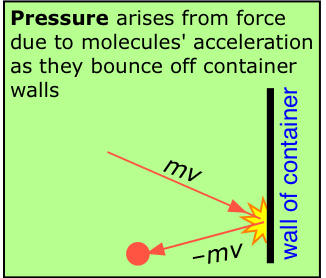At each collision, a molecule moving with momentum mv strikes the surface. Since the collisions are elastic, the molecule bounces back with the same velocity in the opposite direction. This change in velocity ΔV is equivalent to an acceleration a; according to Newton's second law, a force f = ma is thus exerted on the surface of area A exerting a pressure P = f/A.

### Kinetic Interpretation of Temperature

According to the kinetic molecular theory, the average kinetic energy of an ideal gas is directly proportional to the absolute temperature. Kinetic energy is the energy a body has by virtue of its motion:

$K.E. = \dfrac{mv^2}{2}$

As the temperature of a gas rises, the average velocity of the molecules will increase; a doubling of the temperature will increase this velocity by a factor of four. Collisions with the walls of the container will transfer more momentum, and thus more kinetic energy, to the walls. If the walls are cooler than the gas, they will get warmer, returning less kinetic energy to the gas, and causing it to cool until thermal equilibrium is reached. Because temperature depends on the average kinetic energy, the concept of temperature only applies to a statistically meaningful sample of molecules. We will have more to say about molecular velocities and kinetic energies farther on.

• Kinetic explanation of Boyle's law: Boyle's law is easily explained by the kinetic molecular theory. The pressure of a gas depends on the number of times per second that the molecules strike the surface of the container. If we compress the gas to a smaller volume, the same number of molecules are now acting against a smaller surface area, so the number striking per unit of area, and thus the pressure, is now greater.

• Kinetic explanation of Charles' law: Kinetic molecular theory states that an increase in temperature raises the average kinetic energy of the molecules. If the molecules are moving more rapidly but the pressure remains the same, then the molecules must stay farther apart, so that the increase in the rate at which molecules collide with the surface of the container is compensated for by a corresponding increase in the area of this surface as the gas expands.

• Kinetic explanation of Avogadro's law: If we increase the number of gas molecules in a closed container, more of them will collide with the walls per unit time. If the pressure is to remain constant, the volume must increase in proportion, so that the molecules strike the walls less frequently, and over a larger surface area.

• Kinetic explanation of Dalton's law: "Every gas is a vacuum to every other gas". This is the way Dalton stated what we now know as his law of partial pressures. It simply means that each gas present in a mixture of gases acts independently of the others. This makes sense because of one of the fundamental tenets of KMT theory that gas molecules have negligible volumes. So Gas A in mixture of A and B acts as if Gas B were not there at all. Each contributes its own pressure to the total pressure within the container, in proportion to the fraction of the molecules it represents.

## Some important practical applications of KMT

The molecules of a gas are in a state of perpetual motion in which the velocity (that is, the speed and direction) of each molecule is completely random and independent of that of the other molecules. This fundamental assumption of the kinetic-molecular model helps us understand a wide range of commonly-observed phenomena.

## Diffusion: random motion with direction

Diffusion refers to the transport of matter through a concentration gradient; the rule is that substances move (or tend to move) from regions of higher concentration to those of lower concentration. The diffusion of tea out of a tea bag into water, or of perfume from a person, are common examples; we would not expect to see either process happening in reverse!When the stopcock is opened, random motions cause each gas to diffuse into the other container. After diffusion is complete (bottom), individual molecules of both kinds continue to pass between the flasks in both directions.

It might at first seem strange that the random motions of molecules can lead to a completely predictable drift in their ultimate distribution. The key to this apparent paradox is the distinction between an individual and the population. Although we can say nothing about the fate of an individual molecule, the behavior of a large collection ("population") of molecules is subject to the laws of statistics. This is exactly analogous to the manner in which insurance actuarial tables can accurately predict the average longevity of people at a given age, but provide no information on the fate of any single person.

### Effusion and Graham's law

If a tiny hole is made in the wall of a vessel containing a gas, then the rate at which gas molecules leak out of the container will be proportional to the number of molecules that collide with unit area of the wall per second, and thus with the rms - average velocity of the gas molecules. This process, when carried out under idealized conditions, is known as effusion.

Around 1830, the English chemist Thomas Graham (1805-1869) discovered that the relative rates at which two different gases, at the same temperature and pressure, will effuse through identical openings is inversely proportional to the square root of its molar mass.

$v \propto \dfrac{1}{\sqrt{M}}$

Graham's law, as this relation is known, is a simple consequence of the square-root relation between the velocity of a body and its kinetic energy.

$HCl + NH_3 \rightarrow NH_4 Cl_{(s)}$

According to the kinetic molecular theory, the molecules of two gases at the same temperature will possess the same average kinetic energy. If v1and v2 are the average velocities of the two kinds of molecules, then at any given temperature KE1 = KE2 and

$\dfrac{m_1v_1^2}{2} = \dfrac{m_2v_2^2}{2}$

or, in terms of molar masses $$M$$,

$\color{red} { \dfrac{v_1}{v_2} = \sqrt{\dfrac{M_2}{M_1}}}$

Thus the average velocity of the lighter molecules must be greater than those of the heavier molecules, and the ratio of these velocities will be given by the inverse ratio of square roots of the molecular weights. Although Graham's law applies exactly only when a gas diffuses into a vacuum, the law gives useful estimates of relative diffusion rates under more practical conditions, and it provides insight into a wide range of phenomena that depend on the relative average velocities of molecules of different masses.

Example 6.4.1

The glass tube shown above has cotton plugs inserted at either end. The plug on the left is moistened with a few drops of aqueous ammonia, from which $$NH_3$$ gas slowly escapes. The plug on the right is similarly moisted with a strong solution of hydrochloric acid, from which gaseous $$HCl$$ escapes. The gases diffuse in opposite directions within the tube; at the point where they meet, they combine to form solid ammonium chloride, which appears first as a white fog and then begins to coat the inside of the tube.The reaction is

$NH_{3(g)} + HCl_{(g)} \rightarrow NH_4Cl_{(s)}$

1. In what part of the tube (left, right, center) will the NH4Cl first be observed?
2. If the distance between the two ends of the tube is 100 cm, how many cm from the left end of the tube will the NH4Cl first form?

SOLUTION

a) The lighter ammonia molecules will diffuse more rapidly, so the point where the two gases meet will be somewhere in the right half of the tube.

b) The ratio of the diffusion velocities of ammonia (v1)and hydrogen chloride (v2) can be estimated from Graham's law:

$\dfrac{v_1}{v_2} = \sqrt{\dfrac{36.5}{17}} = 1.46$

We can therefore assign relative velocities of the two gases as $$v_1 = 1.46$$ and $$v_2 = 1$$. Clearly, the meeting point will be directly proportional to v1. It will, in fact, be proportional to the ratio v1/(v1+v2)*:

$\dfrac{v_1}{v_1+v_2} \times 100\; cm = \dfrac{1.46}{1.46 + 1.00} \times 100\, cm = 59 \;cm$

*In order to see how this ratio was deduced, consider what would happen in the three special cases in which v1=0, v2=0, and v1=v2, for which the distances (from the left end) would be 0, 50, and 100 cm, respectively. It should be clear that the simpler ratio v1/v2 would lead to absurd results.

Note that the above calculation is only an estimate. Graham's law is strictly valid only under special conditions, the most important one being that no other gases are present. Contrary to what is written in some textbooks and is often taught, Graham's law does not accurately predict the relative rates of escape of the different components of a gaseous mixture into the outside air, nor does it give the rates at which two gases will diffuse through another gas such as air. See Misuse of Graham's Laws by Stephen J. Hawkes, J. Chem. Education 1993 70(10) 836-837

### Uranium enrichment

One application of this principle that was originally suggested by Graham himself but was not realized on a practical basis until a century later is the separation of isotopes. The most important example is the enrichment of uranium in the production of nuclear fission fuel.The K-25 Gaseous Diffusion Plant was one of the major sources of enriched uranium during World War II. It was completed in 1945 and employed 12,000 workers. Owing to the secrecy of the Manhattan Project, the women who operated the system were unaware of the purpose of the plant; they were trained to simply watch the gauges and turn the dials for what they were told was a "government project".Uranium consists mostly of U238, with only 0.7% of the fissionable isotope U235. Uranium is of course a metal, but it reacts with fluorine to form a gaseous hexafluoride, UF6. In the very successful gaseous diffusion process the UF6 diffuses repeatedly through a porous wall. Each time, the lighter isotope passes through a bit more rapidly then the heavier one, yielding a mixture that is minutely richer in U235. The process must be over a thousand times to achieve the desired degree of enrichment. The development of a large-scale diffusion plant was a key part of the U.S. development of the first atomic bomb in 1945. This process is now obsolete, having been replaced by other methods.

### Density fluctuations: Why is the sky blue?

Diffusion ensures that molecules will quickly distribute themselves throughout the volume occupied by the gas in a thoroughly uniform manner. The chances are virtually zero that sufficiently more molecules might momentarily find themselves near one side of a container than the other to result in an observable temporary density or pressure difference. This is a result of simple statistics. But statistical predictions are only valid when the sample population is large.Consider what would happen if we consider extremely small volumes of space: cubes that are about 10–7 cm on each side, for example. Such a cell would contain only a few molecules, and at any one instant we would expect to find some containing more or less than others, although in time they would average out to the same value. The effect of this statistical behavior is to give rise to random fluctuations in the density of a gas over distances comparable to the dimensions of visible light waves. When light passes through a medium whose density is non-uniform, some of the light is scattered. The kind of scattering due to random density fluctuations is called Rayleigh scattering, and it has the property of affecting (scattering) shorter wavelengths more effectively than longer wavelengths. The clear sky appears blue in color because the blue (shorter wavelength) component of sunlight is scattered more. The longer wavelengths remain in the path of the sunlight, available to delight us at sunrise or sunset.[source]

What we have been discussing is a form of what is known as fluctuation phenomena. As the animation shows, the random fluctuations in pressure of a gas on either side do not always completely cancel when the density of molecules (i.e., pressures) are quite small.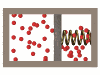Incandescent light bulbs

An interesting application involving several aspects of the kinetic molecular behavior of gases is the use of a gas, usually argon, to extend the lifetime of incandescent lamp bulbs. As a light bulb is used, tungsten atoms evaporate from the filament and condense on the cooler inner wall of the bulb, blackening it and reducing light output. As the filament gets thinner in certain spots, the increased electrical resistance results in a higher local power dissipation, more rapid evaporation, and eventually the filament breaks.

The pressure inside a lamp bulb must be sufficiently low for the mean free path of the gas molecules to be fairly long; otherwise heat would be conducted from the filament too rapidly, and the bulb would melt. (Thermal conduction depends on intermolecular collisions, and a longer mean free path means a lower collision frequency). A complete vacuum would minimize heat conduction, but this would result in such a long mean free path that the tungsten atoms would rapidly migrate to the walls, resulting in a very short filament life and extensive bulb blackening.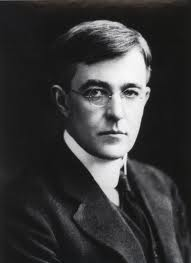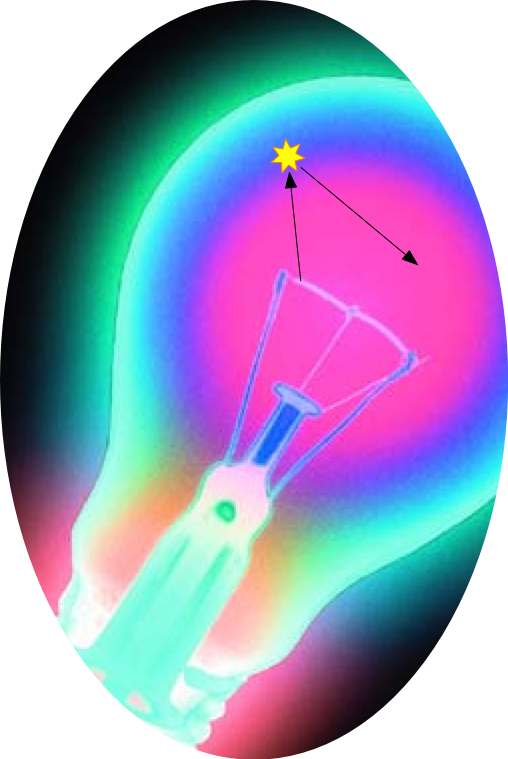Around 1910, the General Electric Company hired Irving Langmuir as one of the first chemists to be employed as an industrial scientist in North America. Langmuir quickly saw that bulb blackening was a consequence of the long mean free path of vaporized tungsten atoms, and he showed that the addition of a small amount of argon will reduce the mean free path, increasing the probability that an outward-moving tungsten atom will collide with an argon atom. A certain proportion of these will eventually find their way back to the filament, partially reconstituting it.

Krypton would be a better choice of gas than argon, since its greater mass would be more effective in changing the direction of the rather heavy tungsten atom. Unfortunately, krypton, being a rarer gas, is around 50 times as expensive as argon, so it is used only in “premium” light bulbs. The more recently-developed halogen-cycle lamp is an interesting chemistry-based method of prolonging the life of a tungsten-filament lamp.

### Viscosity of gases

Gases, like all fluids, exhibit a resistance to flow, a property known as viscosity. The basic cause of viscosity is the random nature of thermally-induced molecular motion. In order to force a fluid through a pipe or tube, an additional non-random translational motion must be superimposed on the thermal motion.

There is a slight problem, however. Molecules flowing near the center of the pipe collide mostly with molecules moving in the same direction at about the same velocity, but those that happen to find themselves near the wall will experience frequent collisions with the wall. Since the molecules in the wall of the pipe are not moving in the direction of the flow, they will tend to absorb more kinetic energy than they return, with the result that the gas molecules closest to the wall of the pipe lose some of their forward momentum. Their random thermal motion will eventually take them deeper into the stream, where they will collide with other flowing molecules and slow them down. This gives rise to a resistance to flow known as viscosity; this is the reason why long gas transmission pipelines need to have pumping stations every 100 km or so.Origin of gas viscosity. This shows the boundary region where random movements of molecules in directions other than the one of the flow (1) move toward the confining surface and temporarily adsorb to it (2). After a short time, thermal energy causes the molecule to be released (4) with most of its velocity not in the flow direction. A rapidly-flowing molecule (3) collides with it (5) and loses some of its flow velocity.

As you know, liquids such as syrup or honey exhibit smaller viscosities at higher temperatures as the increased thermal energy reduces the influence of intermolecular attractions, thus allowing the molecules to slip around each other more easily. Gases, however, behave in just the opposite way; gas viscosity arises from collision-induced transfer of momentum from rapidly-moving molecules to slow ones that have been released from the boundary layer. The higher the temperature, the more rapidly the molecules move and collide with each other, so the higher the viscosity.

### Distribution of gas molecules in a gravitational field

Everyone knows that the air pressure decreases with altitude. This effect is easily understood qualitatively through the kinetic molecular theory. Random thermal motion tends to move gas molecules in all directions equally. In the presence of a gravitational field, however, motions in a downward direction are slightly favored. This causes the concentration, and thus the pressure of a gas to be greater at lower elevations and to decrease without limit at higher elevations.The pressure at any elevation in a vertical column of a fluid is due to the weight of the fluid above it. This causes the pressure to decrease exponentially with height.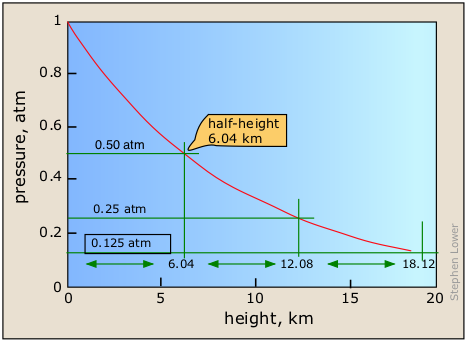This plot shows how the pressure of air at 25° C decreases with altitude. Note the constant increment of altitude (6.04 km, the "half height") required to reduce the pressure by half its value. This reflects the special property of an exponential function a= ey, namely that the derivative da/dy is just ey itself.

The exact functional relationship between pressure and altitude is known as the barometric distribution law. It is easily derived using first-year calculus. For air at 25°C the pressure Ph at any altitude is given by

$P_h = P_o e^{–.011h}$

in which $$P_o$$ is the pressure at sea level.

This is a form of the very common exponential decay law which we will encounter in several different contexts in this course. An exponential decay (or growth) law describes any quantity whose rate of change is directly proportional to its current value, such as the amount of money in a compound-interest savings account or the density of a column of gas at any altitude. The most important feature of any quantity described by this law is that the fractional rate of change of the quantity in question (in this case, ΔP/P or in calculus, dP/P) is a constant. This means that the increase in altitude required to reduce the pressure by half is also a constant, about 6 km in the Earth's case.

Because heavier molecules will be more strongly affected by gravity, their concentrations will fall off more rapidly with elevation. For this reason the partial pressures of the various components of the atmosphere will tend to vary with altitude. The difference in pressure is also affected by the temperature; at higher temperatures there is more thermal motion, and hence a less rapid fall-off of pressure with altitude. Owing to atmospheric convection and turbulence, these effects are not observed in the lower part of the atmosphere, but in the uppermost parts of the atmosphere the heavier molecules do tend to drift downward.

### The ionosphere and radio communication

At very low pressures, mean free paths are sufficiently great that collisions between molecules become rather infrequent. Under these conditions, highly reactive species such as ions, atoms, and molecular fragments that would ordinarily be destroyed on every collision, can persist for appreciable periods of time.

The most important example of this occurs at the top of the Earth's atmosphere, at an altitude of 200 km, at pressure of about 10–7 atm. Here the mean free path will be 107 times its value at 1 atm, or about 1 m. In this part of the atmosphere, known as the thermosphere, the chemistry is dominated by species such as O, O2+ and HO which are formed by the action of intense solar ultraviolet light on the normal atmospheric gases near the top of the stratosphere. The high concentrations of electrically charged species in these regions (sometimes also called the ionosphere) reflect radio waves and are responsible for around-the-world transmission of mid-frequency radio signals.

The ion density in the lower part of the ionosphere (about 80 km altitude) is so great that the radiation from broadcast-band radio stations is absorbed in this region before these waves can reach the reflective high-altitude layers. However, the pressure in this region (known as the D-layer) is great enough that the ions recombine soon after local sunset, causing the D-layer to disappear and allowing the waves to reflect off of the upper (F-layer) part of the ionosphere. This is the reason that distant broadcast stations can only be heard at night.